# MA.912.AR.4.2Export Print
Given a mathematical or real-world context, write and solve one-variable absolute value inequalities. Represent solutions algebraically or graphically.
General Information
Subject Area: Mathematics (B.E.S.T.)
Strand: Algebraic Reasoning
Status: State Board Approved

## Benchmark Instructional Guide

### Terms from the K-12 Glossary

• Absolute Value
• Compound Inequality

### Vertical Alignment

Previous Benchmarks

Next Benchmarks

### Purpose and Instructional Strategies

In grade 6, students solved problems involving absolute value, including context related to distances, temperatures and finances. In middles grades, students solved linear inequalities in one variable. In Algebra I, students solve absolute value inequalities in one variable. In later courses, students will solve one-variable inequalities involving other functions and systems of two-variable inequalities.
• Instruction reinforces the definition of absolute value as a number’s distance from zero (0) on a number line. Distance is expressed as a positive value; example: |3| = 3 and |−3| = 3. The numbers 3 and –3 are each three units away from zero on a number line (MTR.2.1).
• Instruction includes asking for solutions of absolute value equation in word form.
• For example, the equation |$x$| < 3 can be read as “What values of $x$ have absolute value less than 3?” This can be represented algebraically as −3 < $x$ < 3 and graphically.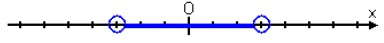• Instruction includes the connection to compound inequalities.
• When solving the inequality |$x$ − 1| ≤ 3, students may set up as $x$ − 1 ≤ 3 and $x$ − 1 ≥ −3.
• When solving the inequality |$x$ − 1| > 3, students may set up as $x$ − 1 > 3 or $x$ − 1 < −3.
• Instruction encourages students to discuss their thinking with their peers (MTR.4.1). Often students will be able to reason out their thinking, but will struggle with representing their thinking mathematically. Encourage this process and ask questions that will help with solving the task (MTR.1.1). Are their multiple ways for students to represent this problem mathematically (MTR.2.1)?

### Common Misconceptions or Errors

• Students may confuse the inequality symbols.
• Students may forget that multiplication or division by a negative number will change the direction of the inequality symbol.
• Students may misrepresent the inequality symbols on a number line and/or incorrectly identify the solutions graphically.
• Students may not create two inequalities from an absolute value inequality.

### Strategies to Support Tiered Instruction

• Teacher models a think-aloud to determine which direction to shade when graphing inequalities.
• For example, “After solving for $x$, I need to select values for $x$. If the value selected creates a true statement, I will place a dot on that value. If it creates a false statement, I will place an “$x$” on that value. In the beginning, I may need to choose a few values before being able to accurately shade. I will replace values for $x$ in both inequalities and then graph solutions to both inequalities on a third number line.”
If |$x$ − 1| ≤ 3, then $x$ − 1 ≤ 3 and $x$ − 1 ≥ −3.
$x$ − 1 ≤ 3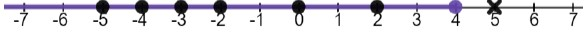$x$ − 1 ≥ −3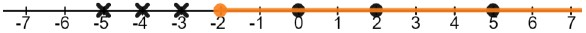|$x$ − 1| ≤ 3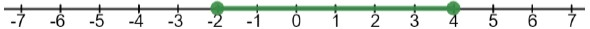• Instruction includes opportunities to create two inequalities from an absolute value inequality using a graphic organizer.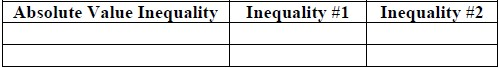• Instruction includes opportunities to create two inequalities from an absolute value inequality using partially worked examples provided in a graphic organizer.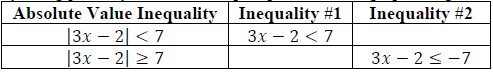• At the Gida Farmhouse Design Company, the average starting salary for a new designer is 35,550, but the actual salary could differ as much as \$1,750 depending on experience. Write an absolute value inequality that could be used to determine the salary range of a new designer.

• Ms. Pruitt’s candy company sells boxes of their famous chocolate-covered peanut butter bites. The candy is sold in boxes that must be within 95 grams of weighing 1,465 grams. Each piece weighs 42.5 grams and the box weighs 116 grams. Calculate and graph the possible quantities of chocolate-covered peanut butter bites that could be in a single box.

• Great Gardens Landscaping has been hired to build gardens around a new resort. They designed plans for two different rectangular gardens. In the first plan the length is twice the length of the width and in the second plan the length is 2 feet longer than the width. The perimeters of the gardens cannot differ more than 3 feet.
• Part A. Write and solve an inequality to determine the range of the widths of the garden.
• Part B. What are the dimensions of the two gardens using the smallest allowable width?
Garden 1: Garden 2:
• Part C. Using the largest allowable width, what is the area of the largest garden?

• Tom is a machinist at Ancient City Choppers. To ensure the highest reliability of the motorcycle engines produced at the shop, Tom machines each cylinder to a 4.01’ diameter with a tolerance of 0.002”.
• Part A. Write an absolute value inequality that could be used to determine the acceptable range of each cylinder’s diameter.
• Part B. Express that range graphically.

### Instructional Items

Instructional Item 1
• There is a cloud layer centered at 4 miles above the sea that is 1,000 feet thick. At what elevations, $x$, given in miles, will a plane in that area be out of the clouds?

Instructional Item 2
• Determine the solutions to the inequality below.
|2$x$ + $\frac{\text{5}}{\text{2}}$| < $\frac{\text{4}}{\text{3}}$

Instructional Item 3
• The thermostat at Jeff’s house is set to 71?, but the temperature may vary by as much as 3°. Write an absolute value inequality to model this situation, and solve for the possible range of temperatures, $t$. Express the solutions both algebraically and graphically.

*The strategies, tasks and items included in the B1G-M are examples and should not be considered comprehensive.

## Related Courses

This benchmark is part of these courses.
1200320: Algebra 1 Honors (Specifically in versions: 2014 - 2015, 2015 - 2022, 2022 and beyond (current))
1200330: Algebra 2 (Specifically in versions: 2014 - 2015, 2015 - 2022, 2022 and beyond (current))
1200340: Algebra 2 Honors (Specifically in versions: 2014 - 2015, 2015 - 2022, 2022 and beyond (current))
1200400: Foundational Skills in Mathematics 9-12 (Specifically in versions: 2014 - 2015, 2015 - 2022, 2022 and beyond (current))
7912095: Access Algebra 2 (Specifically in versions: 2016 - 2018, 2018 - 2019, 2019 - 2022, 2022 and beyond (current))
1200710: Mathematics for College Algebra (Specifically in versions: 2022 and beyond (current))
1209315: Mathematics for ACT and SAT (Specifically in versions: 2022 and beyond (current))

## Related Access Points

Alternate version of this benchmark for students with significant cognitive disabilities.
MA.912.AR.4.AP.2: Solve a one-variable absolute value inequality. Represent solutions algebraically or graphically.

## Related Resources

Vetted resources educators can use to teach the concepts and skills in this benchmark.

## Formative Assessments

Writing Absolute Value Inequalities:

Students are asked to write absolute value inequalities to represent the relationship among values described in word problems.

Type: Formative Assessment

Solving Absolute Value Inequalities:

Students are asked to solve a set of absolute value inequalities.

Type: Formative Assessment

## MFAS Formative Assessments

Solving Absolute Value Inequalities:

Students are asked to solve a set of absolute value inequalities.

Writing Absolute Value Inequalities:

Students are asked to write absolute value inequalities to represent the relationship among values described in word problems.

## Student Resources

Vetted resources students can use to learn the concepts and skills in this benchmark.

## Parent Resources

Vetted resources caregivers can use to help students learn the concepts and skills in this benchmark.